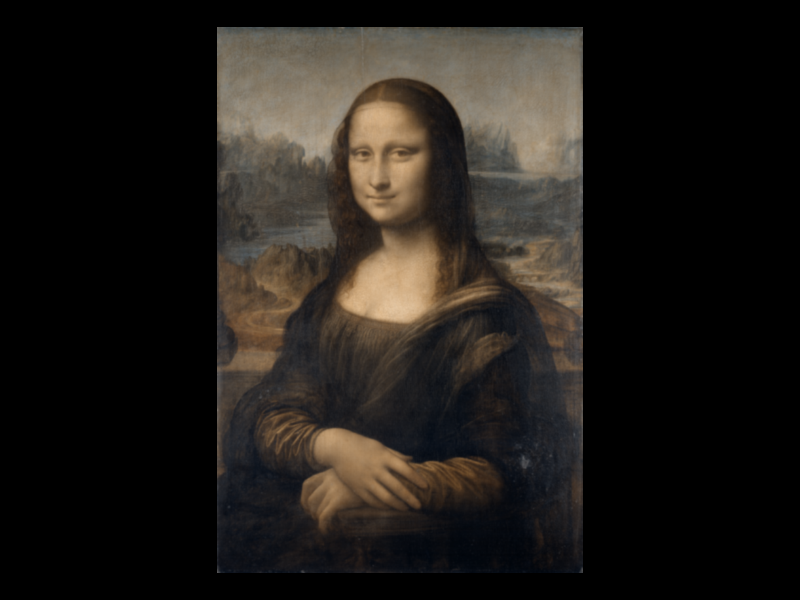# Custom image sampling#

Use custom interpolation kernels for image sampling.

Press k to switch kernel.```import sys
from itertools import cycle

from vispy import scene
from vispy import app

from scipy.signal.windows import gaussian
from scipy.ndimage import gaussian_filter
import numpy as np

canvas = scene.SceneCanvas(keys='interactive')
canvas.size = 800, 600
canvas.show()

# Set up a viewbox to display the image with interactive pan/zoom

# Load the image with a slight blur (so we can later show the sharpening filter)
img_data = gaussian_filter(
sigma=1,
)

# build gaussian kernel
small_gaussian_window = gaussian(5, 1)
small_gaussian_kernel = np.outer(small_gaussian_window, small_gaussian_window)
# normalize
small_gaussian_kernel = small_gaussian_kernel / small_gaussian_kernel.sum()

# do the same but larget and with bigger sigma
big_gaussian_window = gaussian(20, 10)
big_gaussian_kernel = np.outer(big_gaussian_window, big_gaussian_window)
big_gaussian_kernel = big_gaussian_kernel / big_gaussian_kernel.sum()

# sharpening kernel
sharpen_kernel = np.array([[0, -1, 0], [-1, 5, -1], [0, -1, 0]])

kernels = {
'null': np.ones((1, 1)),
'small gaussian': small_gaussian_kernel,
'big gaussian': big_gaussian_kernel,
'sharpening': sharpen_kernel,
}

k_names = cycle(kernels.keys())

k = next(k_names)

image = scene.visuals.Image(
img_data,
interpolation='custom',
custom_kernel=kernels[k],
parent=view.scene,
)

canvas.title = f'Custom sampling with {k} kernel'

# Set 2D camera (the camera will scale to the contents in the scene)
view.camera = scene.PanZoomCamera(aspect=1)
# flip y-axis to have correct aligment
view.camera.flip = (0, 1, 0)
view.camera.set_range()

# Implement key presses
@canvas.events.key_press.connect
def on_key_press(event):
if event.key == 'k':
k = next(k_names)
image.custom_kernel = kernels[k]
canvas.title = f'Custom sampling with {k} kernel'
canvas.update()

if __name__ == '__main__' and sys.flags.interactive == 0:
app.run()
print(__doc__)
```

Total running time of the script: (0 minutes 1.601 seconds)

Gallery generated by Sphinx-Gallery